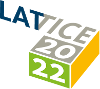#The 39th International Symposium on Lattice Field Theory (Lattice 2022)

Aug 8 – 13, 2022
Hörsaalzentrum Poppelsdorf
Europe/Berlin timezone

## Determination of Lee-Yang edge singularities in QCD by rational approximations

Aug 8, 2022, 2:20 PM
20m
CP1-HSZ/0.011 (CP1-HSZ) - HS1 (CP1-HSZ)

### CP1-HSZ/0.011 (CP1-HSZ) - HS1

#### CP1-HSZ

450
Show room on map
Oral Presentation QCD at Non-zero Density

### Speaker

Kevin Zambello (University of Pisa and INFN - Sezione di Pisa)

### Description

We report updated results on the determination of Lee-Yang edge (LYE) singularities in $N_f=2+1$ QCD using highly improved staggered quarks (HISQ) with physical masses on $N_\tau=4$, $6$, $8$ lattices. The singularity structure of QCD in the complex $\mu_B$ plane is probed using conserved charges calculated at imaginary $\mu_B$. The location of the singularities is determined by studying the (uncancelled) poles of multi-point Padé approximants. We show that close to the Roberge-Weiss (RW) transition, the location of the LYE singularities scales according to the 3-$d$ $\mathbb{Z}_2$ universality class. By combining the new $N_\tau=6$ data with the $N_\tau=4$ data from our previous analysis we extract a rough estimate for the RW temperature in the continuum limit. We also discuss some preliminary results for the singularities close to the chiral phase transition obtained from simulations on $N_\tau=6$, $8$ lattices.

### Primary authors

Kevin Zambello (University of Pisa and INFN - Sezione di Pisa) David Anthony Clarke (Bielefeld University) Jishnu Goswami (RIKEN Center for Computational Science) Guido Nicotra (University of Bielefeld) Christian Schmidt (Bielefeld University) Petros Dimopoulos (University of Parma & INFN) Francesco Di Renzo (Università di Parma and INFN) Simran Singh (University of Parma & INFN)